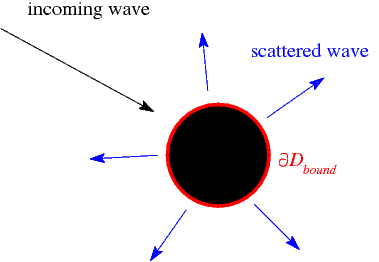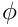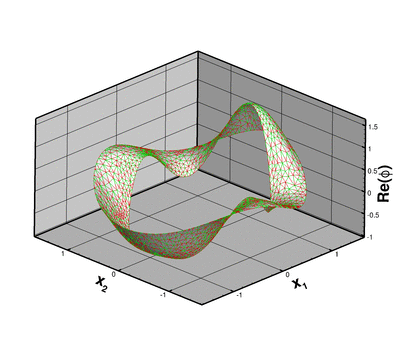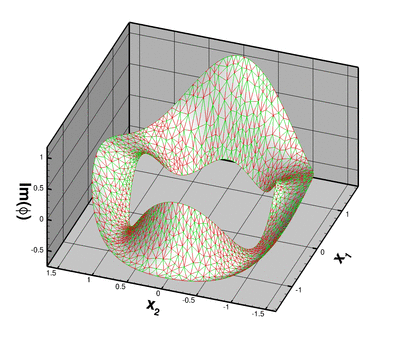Example problem: Solving the Helmholtz equation on an unstructured mesh

In this document we revisit the solution of the Helmholtz equation and demonstrate how to simulate the scattering of a planar wave from a circular cylinder using an unstructured mesh.

# A specific example: Scattering of an acoustic wave from a sound-hard obstacle.

We revisit the problem considered in another tutorial: the scattering of a planar sound wave impacting on a rigid, impermeable obstacle as shown in this sketch:Scattering of an incoming wave from a sound-hard obstacle -- the scatterer.

# Results

The figure below shows plots of the real and imaginary parts of the fluid's displacement potential(a measure of the fluid pressure). The red and green lines correspond to the computed and exact analytical solutions, respectively. (We refer to another tutorial for details.)Real part of the displacement potential (red: computed; green: exact).Imaginary part of the displacement potential (red: computed; green: exact).

# The numerical solution

The driver code for this problem is very similar to the one discussed in another tutorial. Running sdiff on the two driver codes

demo_drivers/helmholtz/scattering/scattering.cc

and

demo_drivers/helmholtz/scattering/unstructured_scattering.cc

shows you the differences, the most important of which are:

• the setup of the unstructured mesh, ensuring that mesh adaptation respects the curvilinear boundaries.

• the re-assignment of element properties following any mesh adaptation – we refer to another tutorial for a more detailed discussion of why this is necessary.

That's all!